## The lcm{1,2,...,n} as a product of values of the sine function sampled over half of the points in a Farey sequence.

Recently Greg Martin (arXiv:0907.4384) showed a cute little formula for the lcm{1,2,3,..,n} = LCM(n).

Jonathan Vos Post reported this on OEIS in A003418 as follows:

"The product of the gamma-function sampled over the set of all rational numbers in the open interval (0, 1) whose denominator in lowest terms is at most n equals ((2*pi)^(1/2))*LCM(n)^(-1/2)."

Thus we look at a Farey sequence. If a/b is a member of this sequence 1−a/b is also a member. We have to compute a product and so we can trade in two Gamma evaluations for one sine evaluation by the reflection formula.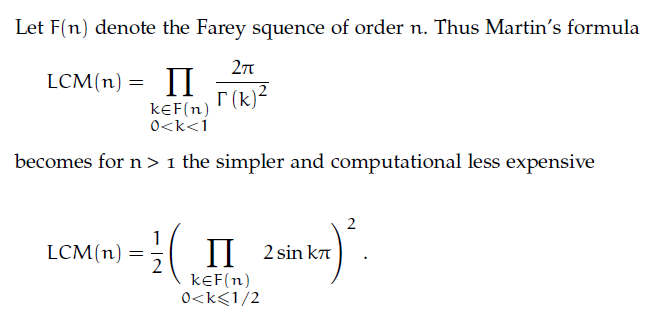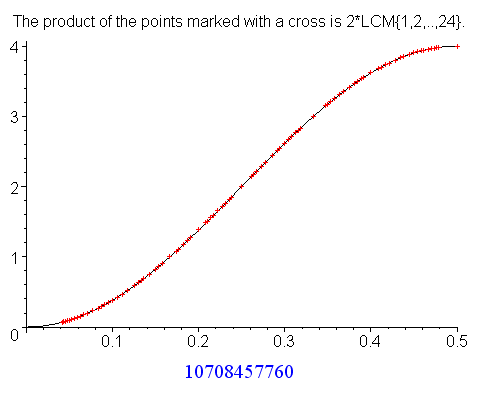## Number theorists like walking the log ...

Therefore let's look at two more items: the number of terms in a Farey sequence of order n, which is Sum{i=1..n} φ(i) − 1 (see A015614). And a nice cup of tea.Here φ(n) denotes Euler's totient function, which is the number of positive integers not exceeding n and relatively prime to n. Further let Λ(n) denote von Mangoldt's function, then the second equation can be rewritten as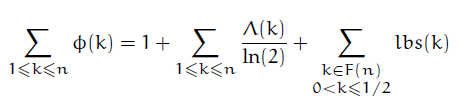The last term, Sum lbs(k), can be approximated by

prox(x) = x( 3x / Pi^2 − 1) − sqrt(x) log(x).

The plot below shows the difference between the true value and the approximation for 3 ≤ n ≤ 3000.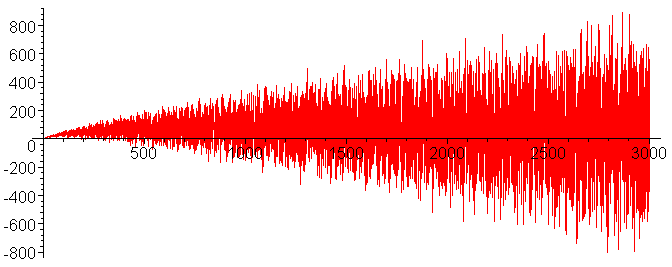See the Wikipedia article for more on Farey sequences.

## Jutta Gut's theorem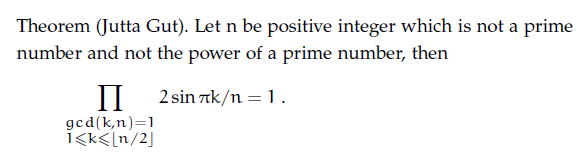### Concerned with sequences:

 OEIS seq Number of fractions in a Farey sequence of order n A005728 1, 2, 3, 5, 7, 11, 13, 19 Sum of phi A002088 0, 1, 2, 4, 6, 10, 12, 18 Lcm of {1,2,3,...n} A003418 1, 1, 2, 6, 12, 60, 60 Lcm of the proper divisors of n A048671 1,1,1, 2,1, 6, 1, 4,3, 10 Exponential von Mangoldt A014963 1, 2, 3, 2, 5, 1, 7, 2, 3 Not a prime power A024619 6, 10, 12, 14, 15, 18, 20 2^phi A066781 2, 2, 4, 4, 16, 4, 64, 16 Prime powers A000961 1, 2, 3, 4, 5, 7, 8, 9 Twice the prime powers A138929 2, 4, 6, 8, 10, 14, 16,18 Cyclotomic polynomials at x = 1 A020500 0, 2, 3, 2, 5, 1, 7, 2, 3 Cyclotomic polynomials at x =-1 A020513 -1, -2,0,1,2,1,3,1,2,1, 5

Here is a Maple worksheet.